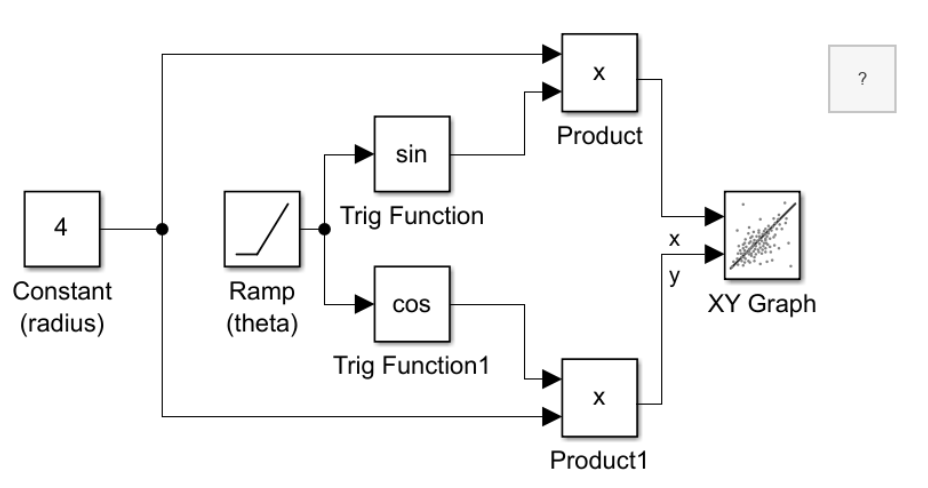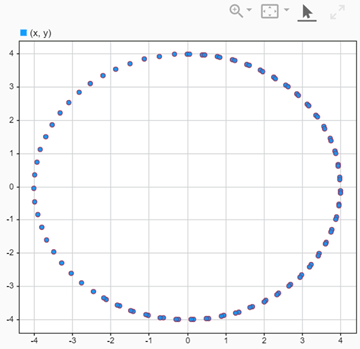Documentation

## Plot Circle with XY Graph

This example plots a circle in Simulink using an XY Graph block.

The `ex_xygraph_block_circle` model computes a circle of radius 4, centered at the origin of x-y plane.The plotted functions are:

``
``

When you simulate the model, a figure window appears showing the plotted circle.

```ans = Simulink.SimulationOutput: tout: [101x1 double] SimulationMetadata: [1x1 Simulink.SimulationMetadata] ErrorMessage: [0x0 char] ```